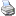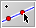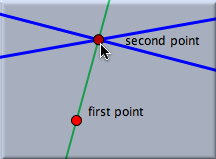backlinks... Add a Circle Add a Line with a Fixed Angle Add a Parallel Add a Perpendicular Add a Point Circle by Radius Copy, Paste, and Macros Distance Gravity Interactive Modes Line through a Point Midpoint Move Mode Multitouch Support Pappus Theorem Rubber Band Segment SegmentJ Spring Table of Contents English 日本語This mode allows a line through two points to be added. The mode is powerful enough to generate the line together with the two points with just one mouse action. When the mouse is pressed, the first point is added. Dragging the mouse generates the second point and the line. When the mouse is released, the position of the second point is frozen, and the construction is finished. The logic behind this mode is very similar to the logic behind other interactive modes, such as add a parallel, add a perpendicular, and add a circle.

Constructing a line in this mode is a three-step procedure:

• Pressing the left mouse button generates the first point. The definition of this point depends on the position of the mouse at the moment the button is pressed:
• If the mouse pointer is over an existing point, then this point is taken as the first point.
• If the mouse pointer is over the intersection of two elements (line, circle, or conic), then this intersection is automatically constructed and taken as the first point.
• If the mouse pointer is over just one element (line or circle), then a point is constructed that is constrained to this element. This point is taken as the first point.
• Otherwise, a free point is added.
• Dragging the mouse generates the line and the second point. The definition of the second point depends on the mouse position. As in add a point mode, the second point snaps to existing elements. The choice of definition is completely analogous to the choice for the first point. The elements that define the second point are always highlighted.
• Releasing the mouse button freezes the definition of the second point. The construction is then finished.

The figures below show the three stages during the construction of a line. Here the first point is a free point and the second point is the intersection of the two existing lines.

 Press the mouse … … drag it … … and release it.#### Synopsis

Add a line mode creates a line connecting two points by a press–drag–release sequence.